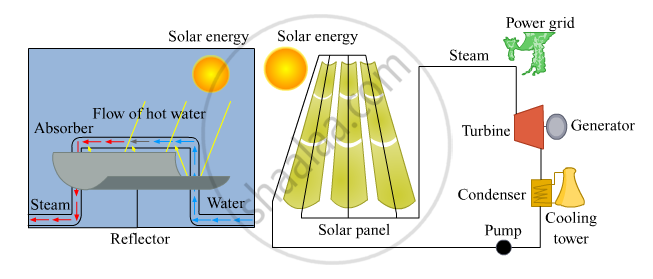# Draw a Schematic Diagram of Solar Thermal Electric Energy Generation. - Science and Technology 2

Draw a schematic diagram of solar thermal electric energy generation.

#### Solution

The schematic diagram of solar thermal electric energy generation is as follows:Concept: Heat Energy (Thermal Energy)
Is there an error in this question or solution?